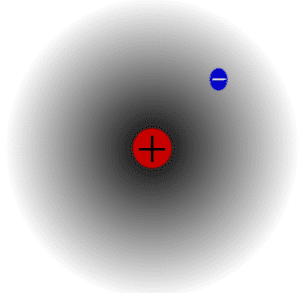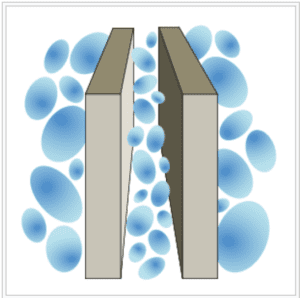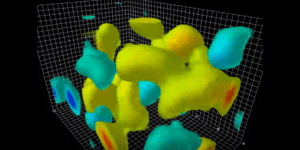Home » renormalization

# renormalization

« Back to Glossary Index

Renormalization is a technique for achieving greater precision in certain physics theories.* In quantum mechanics, renormalization is used to achieve high levels of precision in calculating the amount of mass and charge of subatomic particles like protons. I’ll use the proton as an example.

In the early decades of the 20th century, physicists were trying to calculate the mass and charge of the proton by using the Dirac Equation. The Dirac Equation was developed by British physicist, Paul Dirac, in 1928 as an upgrade of the Schrodinger Equation.

While the Dirac Equation approximated the mass and charge of the proton quite well, when trying for greater precision, the answers devolved into infinity. “Infinity” is not considered by physicists to be an accurate answer because it does not describe anything physical. Everything physical is finite. Further, it was known by measurements taken during experiments that, for example, the charge of a proton is finite rather than infinite.A single proton (red) forms the nucleus of a hydrogen atom. It is positively charged. The single negatively-charged electron is shown in blue. [Image source: Mets501 (Own work); https://en.wikipedia.org/wiki/Proton; CC-BY-SA-3; retrieved Feb. 16, 2018.]It was also known, however, that the measured charge of the proton is only approximate. The measurement is influenced by the behavior of charged particles in the proton’s vicinity. Yet, the physicist wants to calculate the “bare charge” of a proton, that is, the amount of charge possessed by a single proton, uninfluenced by surrounding particles.

Unfortunately, the physicist can’t remove all the surrounding particles because they are virtual. Virtual particles are just like ordinary “real” particles, except that they are extremely short-lived. They pop in and outEphemeral virtual particles in “empty space” between two metal plates create the Casimir Effect. To scale? Forget it. [Image source: https://sv.wikipedia.org/wiki/Casimireffekten]of physical reality from what appears to be empty space. They appear and disappear in the tiniest fractions of a second. At all times, the positively-charged proton is surrounded by a cloud of virtual particles, especially the negatively-charged virtual electrons which are attracted to the proton. There’s nothing that we can do to separate the proton from this cloud.

In addition to wanting to know the charge of a bare proton, physicists also wanted to know that the Dirac Equation is correct. A good check on correctness would be the ability to precisely calculate the charge of a bare proton.

## Renormalization to the RescueRoot beer foam standing in for virtual particles. [Image source: Dr. Don Lincoln, Fermilab;  https://www.fnal.gov/pub/today/archive/ archive_2013/today13-02-01_NutshellReadmore.html]

The solution was renormalization. The technique was developed by three physicists independently: Richard Feynman, Julien Schwinger, and Shinichiro Tomonaga. They shared the Nobel Prize for the achievement in 1965. The Dirac Equation, as upgraded by renormalization and other modifications is called “quantum electrodynamics” or “QED.” It is the current version of that part of quantum mechanics that addresses electromagnetism.

“The foam on a head of root beer is a complicated environment, with bubbles appearing and disappearing in a dizzying display of change. Empty space experiences similar activity, with subatomic particles winking in and out of existence. These ephemeral subatomic particles are real and have a measurable impact on our universe.”–Dr. Don Lincoln, Fermilab, 2013.

In our example, the proton’s charge is renormalized by, first, entering into the equation the amount of the proton’s charge measured by experiment. The measured amount is an approximation rendered imprecise by the influence of virtual particles. Next, the physicist calculates the effects of many of the virtual particles and revises the measurement of proton’s charge accordingly. Bit by bit, the physicist deducts the effects of virtual particles. Today, this is all done by computer, in fact, very long stretches of computer use. The result is a much better approximation of the bare charge of the proton.

Why only an approximation? Two problems. The first is that QED is about quantum behavior, which alwaysSimulation of virtual particles in “empty space” by Professor Derek Leinweber of the University of Adelaide. Click image for video.

include randomness. So, QED calculates probabilities of particular interactions of virtual particles rather than a definite single set of interactions. The result of QED calculations is a range of answers for the bare charge rather than a single, precise answer.

The second problem is that the calculations can’t take into account the effects of all the virtual particles. There is an infinity of possible virtual particle interactions. Each possible interaction modifies the calculation a tiny bit, and when adding an infinity of modifications, the solution to the equation becomes infinity.

Ultimately, however, when the charge of the proton is renormalized, its bare charge can be calculated extremely precisely and agrees with actual measurements. One noted example of the precision of QED is the calculation of the magnetic strength of an electron.** An electron acts like a tiny magnet. By renormalizing, physicists have used computers to calculate the magnetic strength of the electron to 12 decimal places. This is on the order of magnitude of being able to calculate the size of the Atlantic Ocean to the accuracy of the width of a human hair. And the calculated number perfectly matches experimental measurements.

## Renormalization of Other Quantum Theories

QED is the theory of the quantum interactions of matter and light (electromagnetism). Current quantum theory also includes the mathematics of the Strong Force (quantum chromodyamics) and the Weak Force (occasionally called “quantum flavordynamics”). Renormalization is used to gain precision not only in QED, but also in the theories of the Strong Force and Weak Force. These theories are collectively called “quantum field theories.”

## Renormalization Suggests We Have More to Learn

Renormalization results in greater precision than any other theory in physics. However, many physicists, including Richard Feynman, himself, have complained that it’s something of a cheat to put the measured values into the equations “by hand.” They feel that if they truly understood quantum behavior, they would be able to calculate the values rather than entering measured values.

In all, the calculations done in QED require that 19 numbers must be inserted from actual measurements. This includes the amount of electrical charge of a proton as well as its mass. The charges of other particles and the masses of almost all the other particles must also be inserted by hand based on experimental measurements.

Physicists feel that if they truly understood quantum behavior, they would be able to calculate the mass and charge of all particles without having to fall back on measurements. The need to renormalize QED suggests that there may be a deeper, more fundamental theory of quantum behavior. This is one of the motivations for work on string theory.

*In physics, a theory is not just a set of ideas, expressed verbally, as to how something works. Instead, it’s a mathematical equation or equations that describe an aspect of the behavior of the physical universe. For example, the Shrodinger Equation and related equations such as the Born Rule made up the theory of quantum mechanics in the 1920’s.

**The technical term for magnetic strength is “magnetic moment.”

This site uses Akismet to reduce spam. Learn how your comment data is processed.